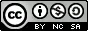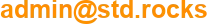# Calculate extra hours with bash script

Here is a bash script which is able to calculate time worked. It takes some arguments (as departure time, arrival time etc..) and gives you back the time spent.

## Code

```#! /bin/bash

#Functions
FunctionGetTime()
{
read -e -p "Arrival time (h:mm) :" -i "8:00" r_HOUR_ARR #get arrival time, 8:00 will be proposed by default
read -e -p "Lunch break (h:mm) :" -i "0:00" r_BREAK_LUN #get lunch break, 0:00 will be proposed by default
read -e -p "Departure time (h:mm) :" -i "17:00" r_HOUR_DEP #get departure time, 17:00 will be proposed by default
if [ "\$1" = false ]; then
read -e -p "Arrival time (to home) (h:mm) :" -i "17:00" r_HOUR_ARR_DOM #get arrival at home, 17:00 will be proposed by default
fi
}

read -e -p "Departure time (from home) (h:mm) :" -i "0:00" r_HOUR_DEP_DOM #get departure time, 0:00 will be proposed by default

if [ "\$r_HOUR_DEP_DOM" == "0:00" ]; then #we don't calculate total time if Departure time is equal to 0:00
echo "We only calculate the intervention time and any overtime."
FunctionGetTime true
else
FunctionGetTime false
fi

#We convert every hour to the universal format (-u to avoid hours differences if executed from another time zone) compared to the time of ref 01/01/1970
HOUR_SOURCE=\$(date -u -d "1970-01-01" +%s)
HOUR_DEP_DOM=\$(date -u -d "1970-01-01 \$r_HOUR_DEP_DOM" +%s)
HOUR_ARR=\$(date -u -d "1970-01-01 \$r_HOUR_ARR" +%s)
BREAK_LUN=\$(date -u -d "1970-01-01 \$r_BREAK_LUN" +%s)
HOUR_DEP=\$(date -u -d "1970-01-01 \$r_HOUR_DEP" +%s)
HOUR_ARR_DOM=\$(date -u -d "1970-01-01 \$r_HOUR_ARR_DOM" +%s)

dej_diff=\$(( (BREAK_LUN - HOUR_SOURCE) ))
duration_diff=\$(( (HOUR_DEP - HOUR_ARR - dej_diff) ))
duration_dest=\$(date -u -d"@\$duration_diff" "+%H:%M")

IN=\$(echo "\$duration_dest" | awk -F: '{ print (\$1 * 60) + \$2 }')

#Extra hours calculation
HOUR_SUPP=\$(date -u -d "1970-01-01 7:00" +%s)
HOUR_SUPP=\$(( (HOUR_SUPP - HOUR_SOURCE) )) #we put 25200 in HOUR_SUPP which is our 7 hours work
duration_diff_sup=\$(( (HOUR_ARR_DOM - HOUR_DEP_DOM - dej_diff) ))

if [ "\$duration_diff_sup" -gt "\$HOUR_SUPP" ]; then #25200 which is our 7 hours work
supp_diff=\$(( (duration_diff_sup - HOUR_SUPP) ))
supp_time=\$(date -u -d"@\$supp_diff" "+%R") #%R is another way to write %H:%M
echo -e "\nCall out time is : \$duration_dest (\$MIN minutes). There is \$supp_time extra hours."
exit 0
elif [ "\$duration_diff" -gt "\$HOUR_SUPP" ]; then
supp_diff=\$(( (duration_diff - HOUR_SUPP) ))
supp_time=\$(date -u -d"@\$supp_diff" "+%R") #%R is another way to write %H:%M
echo -e "\nCall out time is : \$duration_dest (\$MIN minutes). There is \$supp_time extra hours."
exit 0
else
echo -e "\nCall out time is : \$duration_dest (\$MIN minutes). There is no extra hours."
fi

```